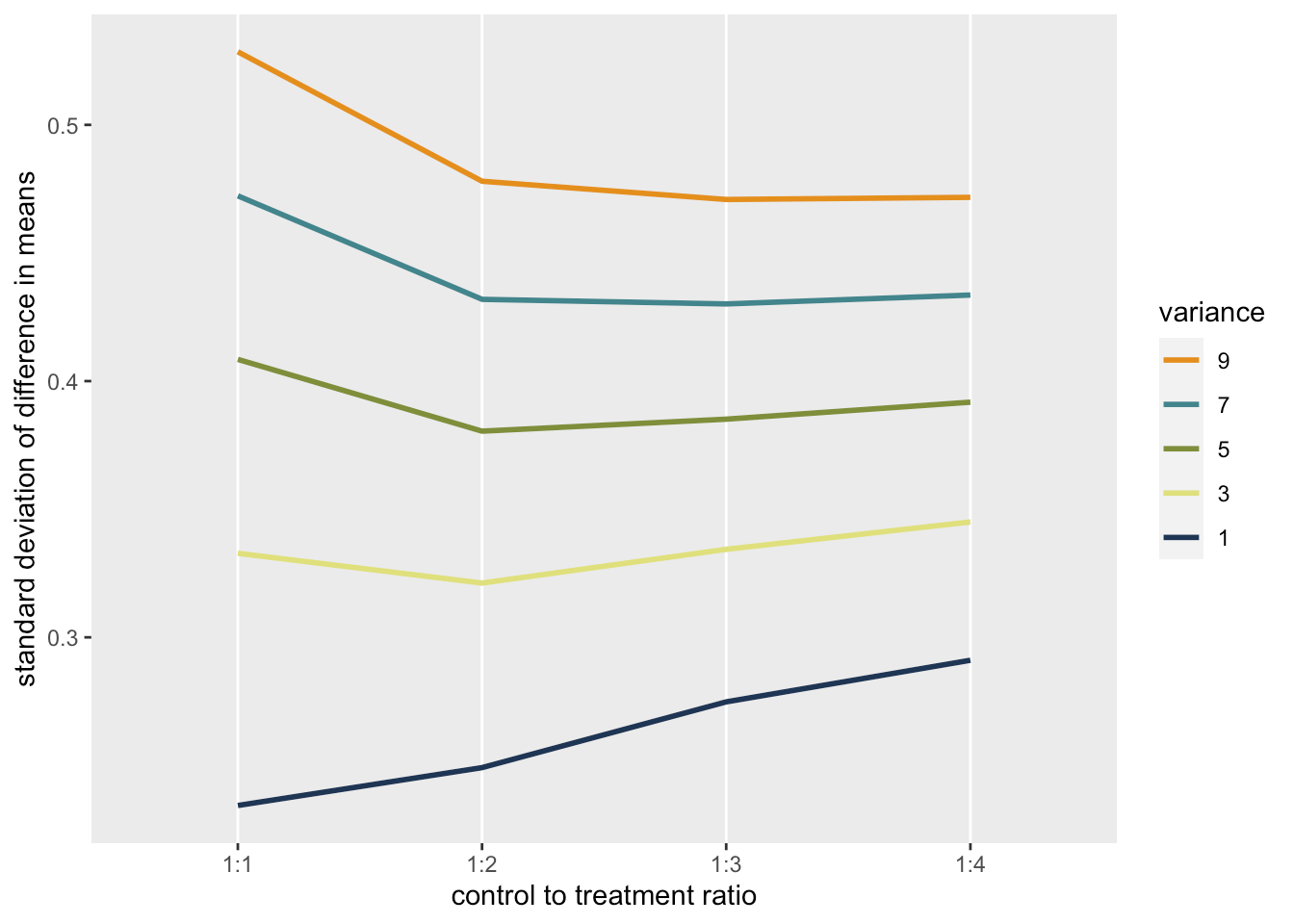Want to share your content on R-bloggers? click here if you have a blog, or here if you don't.

Last time I provided some simulations that suggested that there might not be any efficiency-related benefits to using unbalanced randomization when the outcome is binary. This is a quick follow-up to provide a counter-example where the outcome in a two-group comparison is continuous. If the groups have different amounts of variability, intuitively it makes sense to allocate more patients to the more variable group. Doing this should reduce the variability in the estimate of the mean for that group, which in turn could improve the power of the test.

### Generating two groups with different means and variance

Using `simstudy` (the latest version 1.16 is now available on CRAN), it is possible to generate different levels of variance by specifying a formula in the data definition. In this example, the treatment group variance is five times the control group variance:

```library(simstudy)
library(data.table)

def <- defDataAdd(varname = "y", formula = "1.1*rx",
variance = "1*(rx==0) + 5*(rx==1)", dist = "normal")```

I have written a simple function to generate the data that can be used later in the power experiments:

```genDataSet <- function(n, ratio, def) {

dx <- genData(n)
dx <- trtAssign(dx, grpName = "rx", ratio = ratio)
return(dx[])

}```

And now we can generate and look at some data.

```RNGkind("L'Ecuyer-CMRG")
set.seed(383)

dx1 <- genDataSet(72, c(1, 2), def)
davg <- dx1[, .(avg = mean(y)), keyby = rx]

library(paletteer)
library(ggplot2)

ggplot(data = dx1, aes(x = factor(rx), y = y) ) +
geom_jitter(width = .15, height = 0, aes(color = factor(rx))) +
geom_hline(data= davg, lty = 3, size = .75,
aes(yintercept = avg, color = factor(rx))) +
theme(panel.grid.minor.y = element_blank(),
panel.grid.major.y = element_blank(),
panel.grid.major.x = element_line(color = "grey98"),
legend.position = "none",
axis.title.x = element_blank()) +
scale_x_discrete(labels = c("control", "treatment")) +
scale_color_paletteer_d("jcolors::pal5")```### Power analyses

The following function generates a data set, records the difference in means for the two groups, and estimates the p-value of a t-test that assumes different variances for the two groups.

```genPvalue <- function(n, ratio, def) {

dx <- genDataSet(n, ratio, def)

mean.dif <- dx[rx == 1, mean(y)] - dx[rx == 0, mean(y)]
p.value <- t.test(y~rx, data = dx)\$p.value

data.table(r = paste0(ratio, ":", ratio), mean.dif, p.value)
}```

In this comparison, we are considering three different designs or randomization schemes. In the first, randomization will be 1 to 1, so that half of the sample of 72 (n = 36) is assigned to the treatment arm. In the second, randomization will be 1 to 2, so that 2/3 of the sample (n=48) is assigned to treatment. And in the last, randomization will be 1 to 3, where 3/4 of the patients (n = 54) will be randomized to treatment. For each scenario, we will estimate the mean difference between the groups, the standard deviation of differences, and the power. All of these estimates will be based on 5000 data sets each, and we are still assuming treatment variance is five times the control variance.

```library(parallel)

ratios <- list(c(1, 1), c(1, 2), c(1, 3))

results <- mclapply(ratios, function(r)
rbindlist(mclapply(1:5000, function(x) genPvalue(72, r, def )))
)

results <- rbindlist(results)```

All three schemes provide an unbiased estimate of the effect size, though the unbalanced designs have slightly less variability:

```results[, .(avg.difference = mean(mean.dif),
sd.difference = sd(mean.dif)), keyby = r]
##      r avg.difference sd.difference
## 1: 1:1            1.1          0.41
## 2: 1:2            1.1          0.38
## 3: 1:3            1.1          0.39```

The reduced variability translates into improved power for the unbalanced designs:

```results[, .(power = mean(p.value < 0.05)), keyby = r]
##      r power
## 1: 1:1  0.75
## 2: 1:2  0.81
## 3: 1:3  0.81```

### Benefits of imbalance under different variance assumptions

It seems reasonable to guess that if the discrepancy in variance between the two groups is reduced, there will be less advantage to over-allocating patients in the treatment arm. In fact, it may even be a disadvantage, as in the case of a binary outcome. Likewise, as the discrepancy increases, increased enthusiasm for unbalanced designs may be warranted.

Here is a plot (code available upon request) showing how the variation in the mean differences (shown as a standard deviation) relate to the design scheme and the underlying difference in the variance of the control and treatment groups. In all cases, the assumed variance for the control group was 1. The variance for the treatment group ranged from 1 to 9 in different sets of simulations. At each level of variance, four randomization schemes were evaluated: 1 to 1, 1 to 2, 1 to 3, and 1 to 4.

When variances are equal, there is no apparent benefit to using any other than a 1:1 randomization scheme. Even when the variance of the treatment group increases to 3, there is little benefit to a 1:2 arrangement. At higher levels of variance - in this case 5 - there appears to be more of a benefit to randomizing more people to treatment. However, at all the levels shown here, it does not look like anything above 1:2 is warranted.

So, before heading down the path of unbalanced randomization, make sure to take a look at your variance assumptions.